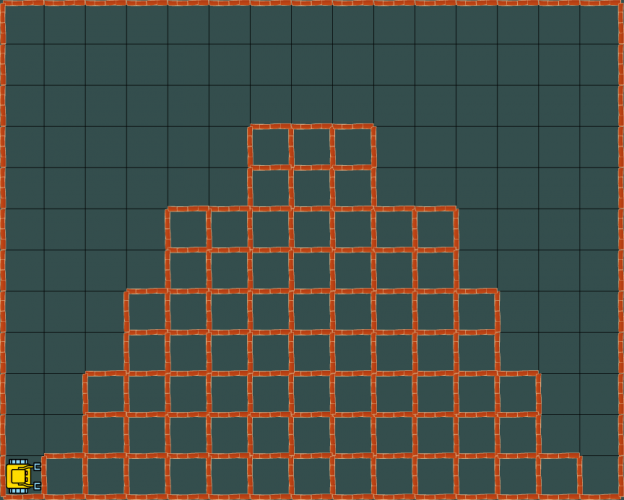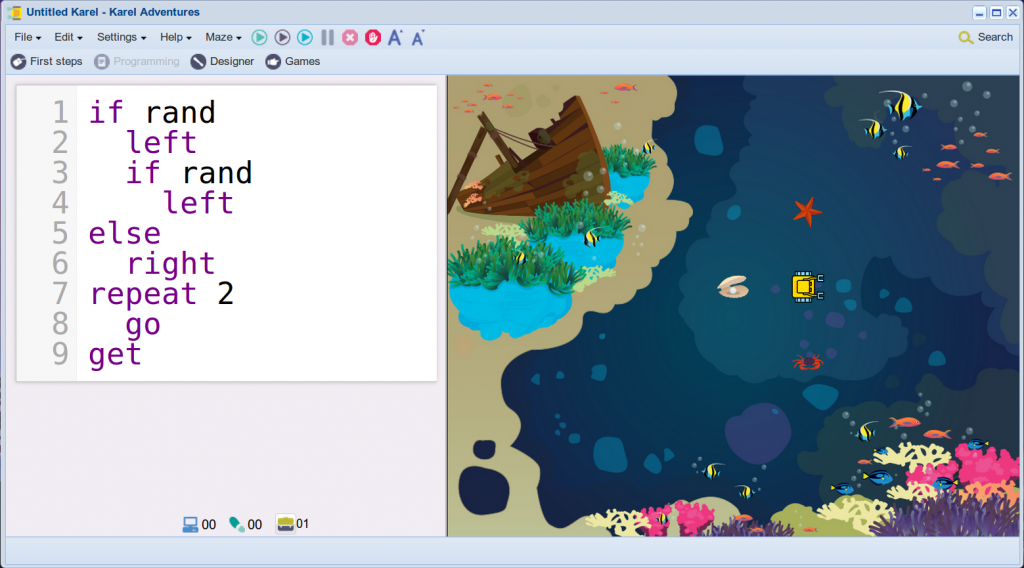# Karel #4: Model with Mathematics

## What It Means

Use math to solve real-world problems, organize data, and understand the world around you.

## Computer Programming Prepares Students for Solving Real-World Problems

The Karel programming course in NCLab provides many opportunities for students to learn and practice real world skills from various parts of Mathematics. Let’s mention just two examples.

### Area Under a Curve

Karel has a GPS device, represented by the commands “gpsx” and “gpsy”. These commands return the horizontal and vertical coordinates of the robot in the maze, respectively. The Southwest corner of the maze has coordinates (0, 0) and the Northeast corner (14, 11). In the game “Lost City”, students are asked to write a program for the robot to count the number of bricks (squares) that form a Mayan pyramid. The shape of the pyramid is random.In reality, students are calculating the area under a curve that is represented by the contour of the pyramid (otherwise called “definite integral”).

### Probability

Karel knows the “rand” command which returns with a 50% chance either True or False. This is a great tool to help students understand probability. For example, with the program below, Karel has a 50% chance to pick up the crab, 25% chance to pick up the starfish, and 25% chance to pick up the pearl. Let the students explain. Ask them whether it is a coincidence that the three probabilities add up to 100%. Let them run the program 100 times and count how many times the robot gets each object. Ask them how is it possible that the crab was not picked up exactly 50 times. The possibilities here are endless…Analogously, one can easily venture into statistics – remember that the robot will not get tired repeating something 500 times!